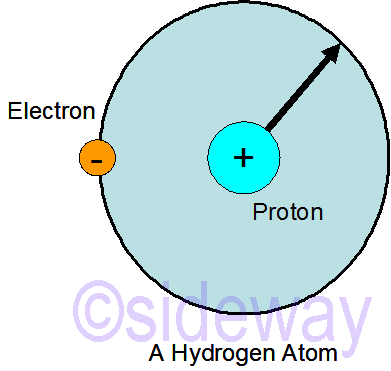output.to from Sideway
Draft for Information Only

# Content

`Electrical Force Charged Particles Electric Field of Spherical shell Electric Dipole  Dipole Electric Field Source and Reference`

# Electrical Force

The electrical forces of magnets and electricity are either induced of generated. These forces work very differently from general mechanic forces, e.g. gravitational forces. In fact, the attractive electrostatic force between two charged particles is far stronger than the gravitation forces being exerted between two solid particles.

## Charged Particles

The tiny particles prsent in atoms are called protons and electrons. Protons are positively charged, while electrons are negatively charged. Both protons and electrons are the smallest existing charged particles. Protons are very tightly packed within a nucleus of an atom. However, electrons can move freely around the nucleus since these electrons are not perpetually attracted to the nucleus. For example a hydrogen atom.The gravity of two masses is equal to ```𝐹grav=𝐺𝑚1𝑚2𝑟2𝑟 𝑚proton=1.7×10−27𝑘𝑔 ; 𝑚electron=9×10−31𝑘𝑔𝑟=5.3×10−11𝑚 ; 𝐺=6.7×10−11𝑁𝑚2𝑘𝑔2 𝐹grav=6.7×10−11(1.7×10−27)(9×10−31)5.3×10−11≈𝑂(10−46)𝑁``` Fhe electricity of two charged particles is ```𝐹elec=14𝜋𝜀0𝑄1𝑄2𝑟2𝑟 𝑒=1.6×10−19𝐶𝑟=5.3×10−11𝑚 ; 14𝜋𝜀0=8.99×109𝑁𝑚2𝐶2 𝐹elec=8.99×109(1.6×10−19)25.3×10−11≈𝑂(10−7)𝑁``` Therefore with reference to an hydrogen atom, the electricity is much stronger than gravity. The ratio of two forces is `𝐹elec𝐹grav≈2.27×1039`

## Electric Field of Spherical shell

For a point particle, when the radius 𝑟 of electric field approaches 0, 𝐸 is undefined at the origin, since 𝑟 is self-contradictory. Therefore the particle cannot exert a force on itself.
For a uniformly charged spherical shell as belowThe charged spherical cell acts like a point particle for 𝑟≫𝑅, i.e. `𝐸𝑠𝑝ℎ𝑒𝑟𝑒=14𝜋𝜀0𝑄𝑟2𝑟; for 𝑟>𝑅 i.e. outside the spherical shell` Due to spherical symmetry, the field is in the 𝑟 direction even close up to the sphere. The total electric field 𝐸𝑠𝑝ℎ𝑒𝑟𝑒 can be calculated using the principle of superposition where 𝐸𝑡𝑜𝑡𝑎𝑙 is the vector sum of the electric field from all charges.
However, the electric field inside the shell is always equal to zero. i.e. `𝐸𝑠𝑝ℎ𝑒𝑟𝑒=0; for 𝑟<𝑅 i.e. inside the spherical shell`

## Electric Dipole

A electric dipole is a pair of two equal but opposite point charges at a distance apart.The dipole moment is `𝑝=𝑞𝑠`

### Dipole Electric Field

By choicing the midpoint of the dipole as the origin, the dipole electric field can be determined as belowThe dipole electric field is equal to ```𝐸0,𝑥=𝐸+,𝑥+𝐸−,𝑥=14𝜋𝜀0𝑞𝑟2+,𝑥𝑟+14𝜋𝜀0−𝑞𝑟2−,𝑥𝑟 𝐸0,𝑥=14𝜋𝜀0𝑞(𝑟−𝑠/2)2+14𝜋𝜀0−𝑞(𝑟+𝑠/2) along 𝑥-axis 𝐸0,𝑥=14𝜋𝜀0𝑞𝑟2+𝑞𝑟𝑠+𝑞𝑠2/4−𝑞𝑟2+𝑞𝑟𝑠−𝑞𝑠2/4(𝑟−𝑠/2)2(𝑟+𝑠/2)2 𝐸0,𝑥=14𝜋𝜀02𝑞𝑟𝑠(𝑟−𝑠/2)2(𝑟+𝑠/2)2 ``` For electric field far away from the electric dipole, i.e 𝑟≫𝑠, then the dipole electric field along 𝑥-axis is ```𝑟−𝑠22≈𝑟+𝑠22≈𝑟2 𝐸0,𝑥=14𝜋𝜀02𝑞𝑠𝑟3 𝐸0,𝑥=14𝜋𝜀02𝑞𝑠𝑟3,0,0 ``` For the electric field of point particle is approximately equal to ~1/𝑟2 and the electric field of dipole is approximately equal to ~1/𝑟3.

## Source and Reference

https://www.youtube.com/watch?v=v57B_1ZBAho&list=PLZ6kagz8q0bvxaUKCe2RRvU_h7wtNNxxi&index=1

©sideway

ID: 191100302 Last Updated: 11/3/2019 Revision: 0Home 5

Business

Management

HBR 3

Information

Recreation

Culture

Chinese 1097

English 337

Computer

Hardware 153

Software

Application 204

Latex 34

Manim 171

Numeric 19

Programming

Web 285

Unicode 504

HTML 65

CSS 59

ASP.NET 194

OS 412

Python 56

Knowledge

Mathematics

Algebra 30

Geometry 21

Calculus 67

Engineering

Mechanical

Rigid Bodies

Statics 92

Dynamics 37

Control

Natural Sciences

Electric 27

Copyright © 2000-2021 Sideway . All rights reserved Disclaimers last modified on 06 September 2019